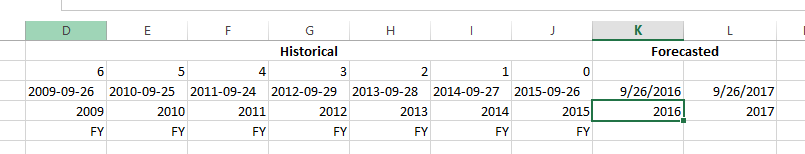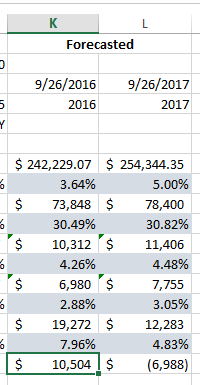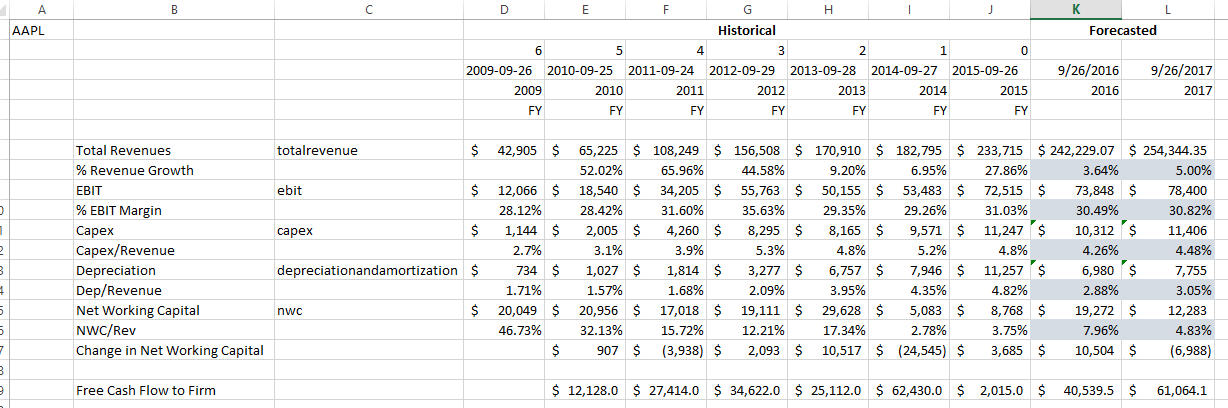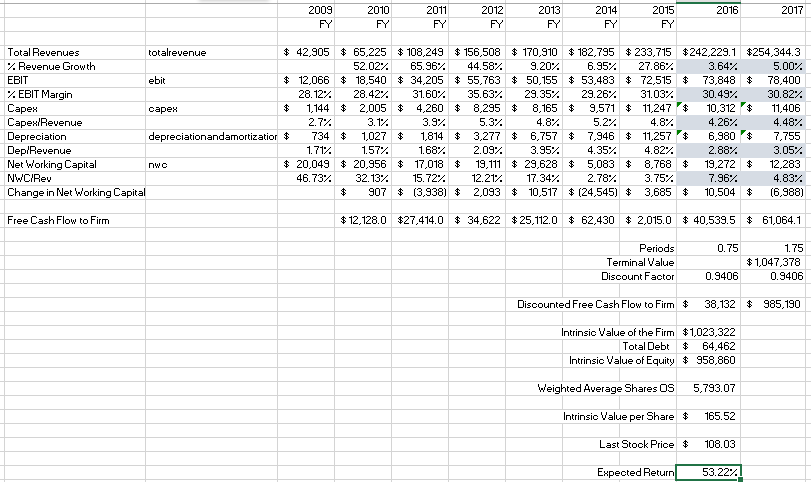# DCF Valuation Course Case Study

## Valuation:

The process of determining the current worth of an asset or company. There are many techniques that can be used to determine value, some are subjective and others are objective.

For example, an analyst valuing a company may look at the company’s management, the composition of its capital structure, prospect of future earnings, and market value of assets.

Judging the contributions of a company’s management would be more of a subjective valuation technique, while calculating intrinsic value based on future earnings would be an objective technique.

For our class with USF, we used the objective approach. We helped the students use Intrinio data to construct a Discounted Cash Flow Model (DCF) in order to determine the intrinsic value of a company.

Like most parts of financial analysis, the hardest part about a DCF valuation is gathering the data necessary to build the model. Without the use of innovative tools like the Intrinio financial data platform, students (and professionals) can be left spending (wasting) hours entering the data before they can actually analyze it and build their model.

##### Instead, using the Intrinio Excel add-in, the students at USF were able to quickly build a dynamic model that saves them time moving forward. If they want to value a new company – they simply change the ticker. Without the Intrinio Excel add-in, they’d need to type each new value in by hand.

It is estimated that Intrinio saved each student at University of South Florida an average of 10 hours of data entry during their studies of Valuation.

Aside from learning the valuable skills of valuation, the students at USF gained an advanced understanding of Excel modeling and learned tips, tricks and shortcuts for getting the most out of the functionality of Excel – very important skills for a future in the business world.

## Installation

The class began by quickly installing the Intrinio Excel add-in on each student’s own personal computer (Mac OS X & Microsoft Windows). The entire install process took less than 5 minutes, and the students followed along with the directions and YouTube video on the Intrinio channel.

Once the Excel add-in was installed on each student’s computer, we walked them through the process of building a DCF step by step.

## Setup

Begin by naming your first Sheet in Excel “DCF” (discounted cash flow), and naming a second sheet “WACC” (weighted average cost of capital).

Choose a ticker and enter it in cell A1 – so that it can be referenced throughout the entire model. In our example, we use AAPL (Apple).

Click on the A1 Cell, then click on the named range box just above it. Delete “A1” and type the word “ticker.” Now, in each of your formulas you’ll be able to simply type “ticker” instead of “AAPL” or “A1.”

Note: Some of your data will look different from the data in this Case Study because it will be more current – the data in the following screenshots and examples is how it looked for the USF students the day they built their DCF.

## Cash Flow Model

Skipping a couple of lines, enter in each item that we will pull in data for and forecast for AAPL in cells B7-B19.

In the next column, next to the actual values (Total Revenues) but not the ratios/percentages (% Revenue Growth), put the associated “Intrinio Tag” that we’ll use to pull the data in. This will make building our formulas much easier.

• totalrevenue
• ebit
• capex
• depreciationandamortization
• nwc

## Historical & Projected Fiscal Years

For this case, we’re going to be pulling in historical data dating back to 2009, and forecasting out two years to 2017. We use something called a “sequence” number in our data feed, which represents the “nth” item in a data series. Since we’re going back 6 years (2015 is sequence number 0), we’ll need 6 items in the sequence.

#### In cell J2, type “0”, then in cell I2 type “J2+1”, then drag across to cell “D2.”

You should have populated 6, 5, 4, 3, 2, 1, 0 across row 2.Next, underneath each of these sequence numbers in row 3, we’ll want to populate the corresponding end-dates for the income statement (the data we’re pulling in).

#### =IntrinioFundamentals(ticker,”income_statement”,”FY”,J2,”end_date”)

It should populate with: 2015-09-26

You can drag this across to sequence number 6 in D3 and the whole row should populate.

By using formulas like this to pull in seemingly simple numbers, we are making the model extremely dynamic and flexible. It may seem simpler to type out 2015-09-26 -> but in fact you’ll be creating a dynamic model and saving time by using the formulas to pull this data in.

Next, underneath these dates in row 4, we’ll want to populate the fiscal year associated with the historical dates and statements (2009-2015).

#### =IntrinioFundamentals(ticker,”income_statement”,”FY”,J2,”fiscal_year”)

It should populate with: 2015

You can drag this across to column D, and the whole row should populate with the correct fiscal years corresponding to the sequence number.Lastly, underneath this we’ll want to pull in the fiscal period for each cell (this is just FY, indicating that these are fiscal year values, as opposed to quarterly, etc.).

#### =IntrinioFundamentals(ticker,”income_statement”,”FY”,H2,”fiscal_period”)

It should populate with: FY

You can drag this across to column D, and the whole row should populate with the correct fiscal periods.

#### Merge and center cells K1 and L1, and type in “Forecasted” to indicate that these columns will have forecasted data.

We want our first forecasted date to be next year on the same month, same day.

#### =DATE(YEAR(J3)+1,MONTH(J3),DAY(J3))

It should populate with: 09/26/2016

You can drag this cell over one into L3 to populate the following year.

Underneath this, we want to pull in the associated years (2016 and 2017).

#### =+J4+1

It should populate with: 2016

You can drag this cell over one into L4 to populate the following year.

#### So far, your DCF workbook should look something like this:## Historical Data

Next we’re going to populate the raw historical data from 2009 – 2015. Using Intrinio formulas we’ll pull in all the historical data for Revenues, EBIT, CAPEX, Depreciation and Net Working Capital.

#### =IntrinioFinancials(ticker,”income_statement”,D4,D5,\$C\$7,”M”)

You’re referencing the ticker, “2009” (D4), “FY” (D5), as well as the associated tag “totalrevenue” (\$C\$7).

\$C\$7 is being hard coded (\$) so that when we drag the formulas it doesn’t auto-populate. This value will change.

“M” stands for millions. You can return values in “A” (actuals), “K” (thousands), “M” (millions) or “B” (billions).

If we were writing the whole formula out by hand, it would look like this:

=IntrinioFinancials(“AAPL”,”income_statement”,2009,”FY”,”totalrevenue”,”M”)

You can click and drag this cell across all the way to J7 and you should see the associated revenue values populate.

#### =IntrinioFinancials(ticker,”calculations”,D4,D5,\$C\$9,”M”)

You’re referencing the ticker, “2009” (D4), “FY” (D5), as well as the associated tag “ebit” (\$C\$9).

If we were writing the whole formula out by hand, it would look like this:

=IntrinioFinancials(“AAPL”,”calculations”,2009,”FY”,”ebit”,”M”)

You can click and drag this cell across all the way to J9 and you should see the associated EBIT values populate.

#### =IntrinioFinancials(ticker,”calculations”,D4,D5,\$C\$11,”M”)

You’re referencing the ticker, “2009” (D4), “FY” (D5), as well as the associated tag “capex” (\$C\$11).

If we were writing the whole formula out by hand, it would look like this:

=IntrinioFinancials(“AAPL”,”calculations”,2009,”FY”,”capex”,”M”)

You can click and drag this cell across all the way to J11 and you should see the associated CAPEX values populate.

#### =IntrinioFinancials(ticker,”calculations”,D4,D5,\$C\$13,”M”)

You’re referencing the ticker, “2009” (D4), “FY” (D5), as well as the associated tag “depreciationandamortization” (\$C\$13).

If we were writing the whole formula out by hand, it would look like this:

=IntrinioFinancials(“AAPL”,”calculations”,2009,”FY”,”depreciationandamortization”,”M”)

You can click and drag this cell across all the way to J13 and you should see the associated capex values populate.

#### =IntrinioFinancials(ticker,”calculations”,D4,D5,\$C\$15,”M”)

You’re referencing the ticker, “2009” (D4), “FY” (D5), as well as the associated tag “nwc” (\$C\$15).

If we were writing the whole formula out by hand, it would look like this:

=IntrinioFinancials(“AAPL”,”calculations”,2009,”FY”,”nwc”,”M”)

You can click and drag this cell across all the way to J15 and you should see the associated capex values populate.

## Historical Ratios

Next we’ll want to use some basic math and formulas to calculate historical ratios based off of the data we just pulled in. For example, we’ll want to know how Revenue has grown over past years in order to predict how it will grow in the future.

To calculate Revenue growth, we’ll want to take the chosen year divided by the previous year minus one. Because this ratio takes into account the previous year to measure growth, we’ll start in 2010 and leave 2009 blank.

#### =E7/D7-1

You can click the % button in the top ribbon bar to make this a percentage, and click and drag this cell across to J8 to populate the rest of the revenue growth numbers.

To calculate % EBIT Margin, we’ll want to take the 2009 EBIT and divide it by the 2009 Total Revenues.

#### =D9/D7

You can click the % button in the top ribbon bar to make this a percentage, and click and drag this cell across to J10 to populate the rest of the % EBIT Margin numbers.

To calculate CAPEX/Revenue, we’ll want to take the 2009 CAPEX and divide it by the 2009 Total Revenues.

#### =D11/D7

You can click the % button in the top ribbon bar to make this a percentage, and click and drag this cell across to J12 to populate the rest of the CAPEX/Revenue numbers.

To calculate Depreciation/Revenue, we’ll want to take the 2009 Depreciation and divide it by the 2009 Total Revenues.

#### =D13/D7

You can click the % button in the top ribbon bar to make this a percentage, and click and drag this cell across to J14 to populate the rest of the Depreciation/Revenue numbers.

To calculate NWC/Revenue, we’ll want to take the 2009 NWC and divide it by the 2009 Total Revenues.

#### =D15/D7

You can click the % button in the top ribbon bar to make this a percentage, and click and drag this cell across to J16 to populate the rest of the Depreciation/Revenue numbers.

To calculate the change in NWC, we’ll want to take the 2010 NWC and subtract the 2009 NWC (since this is a growth number we’ll leave 2009 blank and start in 2010).

#### =E15-D15

You can click and drag this cell across to J17 to populate the rest of the change in NWC numbers.

#### So far, your DCF workbook should look something like this:## Forecasted Values

Next, we want to pull in our forecasts for Revenue, EBIT, CAPEX, Depreciation, and NWC for 2016 and 2017.

To forecast Total Revenues, we’ll use the Intrinio formula for Wall Street consensus Revenues. We get this data from a company called Zacks, which sources it from top Wall Street analysts. We’ll want to divide this value by 1,000,000 to adjust the raw data.

#### =IntrinioDataPoint(ticker,”next_yr_ave_revenue_est”)/1000000

To project % Revenue Growth in 2016, we’ll want to take the Revenue forecast for 2016 and divide by Revenues in 2015 minus 1.

#### =K7/J7-1

You can click and drag this cell across to L8 to project % Revenue Growth for AAPL in 2017.

To forecast EBIT in 2016, we need to first know the % EBIT Margin for 2016. To calculate this, we’ll take the average of all the historical data for % EBIT Margin.

#### =AVERAGE(D10:J10)

You can click and drag this formula across to cell L10 to forecast AAPL’s % EBIT Margin for 2017.

Moving back up a line to EBIT in 2016, we can now forecast this by multiplying Revenues in 2016 by % EBIT Margin in 2016.

#### =K10*K7

You can click and drag this formula across to cell L9 to forecast AAPL’s EBIT for 2017.

Similarly, to calculate CAPEX in 2016 we’ll need to first calculate CAPEX/Revenue for 2016. We do this by taking the average of all the historical data for CAPEX/Revenue.

#### =AVERAGE(D12:J12)

You can click and drag this formula across to cell L12 to forecast AAPL’s CAPEX/Revenue for 2017.

Moving back up a line to CAPEX in 2016, we can now forecast this by multiplying Revenues in 2016 by CAPEX/Revenue in 2016.

#### =K12*K7

You can click and drag this formula across to cell L11 to forecast AAPL’s CAPEX for 2017.

The same goes for Depreciation: we must first calculate Depreciation/Revenue for 2016.

#### =AVERAGE(D14:J14)

You can click and drag this formula across to cell L14 to forecast AAPL’s Depreciation/Revenue for 2017.

Moving back up a line to Depreciation in 2016, we can now forecast this by multiplying Revenues in 2016 by Depreciation/Revenue in 2016.

#### =K14*K7

You can click and drag this formula across to cell L13 to forecast AAPL’s Depreciation for 2017.

Surprise, in order to calculate NWC for 2016 we must first calculate NWC/Revenue in 2016.

#### =AVERAGE(H16:J16)

In this case, we only take the average of the past three years instead of averaging all of the historical data. We do this because NWC/Revenue in years 2009-2012 is substantially higher than the rest of the years (outliers) and that doesn’t fit into a normalized projection for the future – so we don’t want to include it in our calculation of the average.

You can click and drag this formula across to cell L16 to forecast AAPL’s NWC/Revenue for 2017.

Finally, we can calculate NWC for 2016 by multiplying Revenues in 2016 by NWC/Revenue in 2016.

#### =K16*K7

You can click and drag this formula across to cell L15 to forecast AAPL’s NWC for 2017.

To finish off our projections, we’ll project the change in NWC from 2016 to 2017. We do this by subtracting the actual historical value for NWC in 2015 from our projected value for NWC in 2016.

#### =K15-J15

You can click and drag this formula across to cell L17 to forecast AAPL’s change in NWC from 2016 to 2017.

#### Your projections for the DCF should now look something like this:## Weighted Average Cost of Capital

At this point in the model, we’re going to switch over to the WACC worksheet and calculate the weighted average cost of capital before we finish this DCF.

### Setup

In your WACC worksheet, type the following to get set up:

• “DEBT” into cell D4
• “EQUITY” into cell J4
• “WACC” into cell D11

### Cost of Debt

Underneath “Debt”, type the following into cells D5-D7:

• Moody’s AAA Yield
• Tax Rate
• After Tax Cost of Debt

In cell E5, we’ll use the Intrinio formula that pulls in Moody’s AAA Yield from the Federal Reserve. This percentage represents the riskiness of investment grade corporate bonds. In the case of AAPL we’ll use the AAA curve because it represents the highest rated corporates yield to maturity, and AAPL carries a lot of cash and is unlikely to go bankrupt. However, depending on the riskiness of the company you have chosen, you can also pull in the BBB curve.

#### =IntrinioDataPoint(“FRED.AAA”,”value”)/100

We divide by 100 to transform the return value into a percentage.

#### =IntrinioDataPoint(ticker,”efftaxrate”)

To calculate the after-tax cost of debt, we need to take the AAA Yield times (1-tax rate).

#### =E5*(1-E6)

We calculate the cost of debt “after” taxes, because interest expense is tax deductive and dividends paid is not. This means there is a tax advantage to taking out debt.

#### Your Cost of Debt section should look something like this:### Cost of Equity

Underneath “Equity”, type the following into cells J5-J8:

• Risk Free Rate
• Beta
• Cost of Equity

#### In cell K5, we’ll use the Intrinio formula to pull in the risk free rate from the Federal Reserve:

This is the 10-year treasury constant maturity

#### =IntrinioDataPoint(ticker,”beta”)

To calculate the Equity Risk Premium, we use data from Aswath Damodaran a finance professor at the Stern School of Business at New York University. At Intrinio, we call him the Godfather of Value Investing. He is a recognized authoritative source for equity risk premium data.

#### =IntrinioDataPoint(“DMD.ERP”,”ttm_erp”)

Lastly to calculate the Cost of Equity, we’ll take the Risk Free Rate plus (Beta times the Equity Risk Premium).

#### Your Cost of Equity Section should look something like this:#### WACC

Underneath “WACC”, type the following into cells D12-D14:

• Debt
• Equity
• Total Market Value

#### =SUM(E12:E13)

In cell F12, next to the value of debt, we want to calculate debt as a percentage of the total market value.

#### =E12/\$E\$14

In cell F13, next to the value of equity, we want to calculate equity as a percentage of the total market value.

## Free Cash Flow to Firm

Now that we have calculated the WACC for AAPL, we can head back to the DCF page to finish off the Free Cash flows, discount them, and arrive at an intrinsic value.

Back in the DCF worksheet, we need to calculate the historical FCFF (Free Cash Flow to Firm) for years 2009-2015.

The formula to calculate FCFF is the following:

EBIT * (1-Tax Rate) – CAPEX + Depreciation – Change in NWC

#### =E9*(1-IntrinioFinancials(ticker,”calculations”,E4,E5,”efftaxrate”))-E11+E13-E17

You can click and drag this formula across to cell J19 to calculate FCFF for the remaining historical years.

#### =K9*(1-WACC!\$E\$6)-K11+K13-K17

The “WACC!\$E\$6” populates when you reference the effective tax rate from the WACC workbook (cell reference).

You can click and drag this formula across to cell L19 to project FCFF for AAPL for 2017.

#### Your DCF Worksheet should now look something like this:## Discounting Free Cash Flows

The next step after calculating Free Cash Flows is to Discount those Free Cash Flows to their present value. To do this, we need to calculate the discount rate. We’ll need to calculate the time periods for the future cash flow as well as the terminal value (FCFF into perpetuity).

#### In cells J20-J22, type in the following:

• Periods
• Terminal Value
• Discount Factor

#### =(K3-NOW())/365

You can click this cell and drag across to cell L20 to calculate the period value for 2017.

Leaving cell K22 blank, we’ll calculate the Terminal Value in cell L22.

The formula for Terminal Value is the following:

Last Period FCFF / (WACC – long term growth rate)

#### =L19/(WACC!H14-0.02)

WACC!H14 is a cell reference to the WACC value calculated on the WACC workbook.

We use 0.02 as a long term growth rate because economist consensus for long term nominal GDP growth is around 2-3%.

Next we want to calculate the discount factor.

The formula for discount factor is:

(1-r) ^ t

r = rate and t = time periods

#### =(1-WACC!H14)^DCF!K21

You can click and drag this cell across to L22 to calculate the discount factor for 2017.

Next, to calculate the Discounted Free Cash Flow to Firm we want to multiply the Free Cash Flow for each projected year by the associated Discount Rate.

#### The Discounted FCFF section of your DCF worksheet should now look like this:## Calculating Intrinsic Value

Now that we’ve discounted our cash flows, we’re ready to use them to calculate the intrinsic value of AAPL.

In cells J27-J29, type the following:

• Intrinsic Value of the Firm
• Total Debt
• Intrinsic Value of Equity

Next, skipping a row each time, in cells J31, J33, J35 and J37, type the following:

• Weighted Average Shares Outstanding
• Intrinsic Value Per Share
• Last Stock Price
• Expected Return

Intrinsic Value of The Firm is calculated by simply adding the Discounted FCFF for the projected years together.

#### =IntrinioDataPoint(ticker,”weightedavedilutedsharesos”)/1000000

To calculate the intrinsic value per share, we simply take the intrinsic value of equity divided by the weighted average shares outstanding.

#### =IntrinioDataPoint(ticker,”close_price”)

Lastly, to calculate our expected return, we want to take the intrinsic value per share divided by the last stock price minus one.

####Congratulations, you’re done! We’ve just created a comprehensive discounted cash flow valuation for AAPL, calculating an intrinsic value of \$165.52 and an expected return of 53.22%. Obviously, this entire exercise is based on a set of assumptions. Feel free to play around with the model, change some assumptions, and see how it affects the calculated value of the company.

#### In the end, the DCF workbook should look something like this:#### If you are a student or professor interested in Intrinio Financial Data or have questions about this Quant Model exercise, please visit intrinio.com and click the green chat button in the right hand corner of the website.

Get started for free today by visiting www.intrinio.com. Register with your email and check out our documentation or YouTube videos for help getting started.